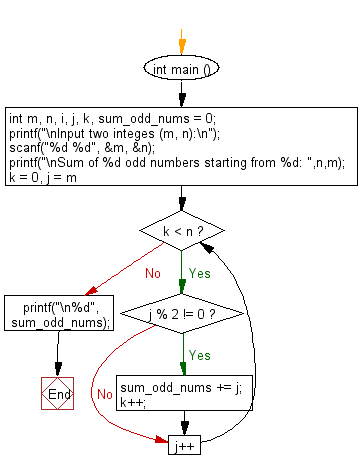﻿ C Program: Sum of n odd numbers starting from m - w3resource

# C Exercises: Sum of n odd numbers starting from m

## C Basic Declarations and Expressions: Exercise-123 with Solution

Write a C program that reads two integers m, n and computes the sum of n odd numbers starting from m.

Sample Solution:

C Code:

``````#include <stdio.h>

int main () {
int m, n, i, j, k, sum_odd_nums = 0;

// Prompt user for input
printf("\nInput two integers (m, n):\n");

// Read two integer values 'm' and 'n' from user
scanf("%d %d", &m, &n);

// Print a message indicating what the program will do
printf("\nSum of %d odd numbers starting from %d: ",n,m);

// Loop to find and sum 'n' odd numbers starting from 'm'
for (k = 0, j = m; k < n; j++) {

// Check if 'j' is odd
if (j % 2 != 0) {
sum_odd_nums += j; // Accumulate odd numbers
k++; // Increment the count of odd numbers found
}
}

// Print the sum of odd numbers
printf("\n%d", sum_odd_nums);

return 0; // End of program
}
``````

Sample Output:

```Input two integes (m, n):
65
5

Sum of 5 odd numbers starting from 65:
345
```

Flowchart:C programming Code Editor:

What is the difficulty level of this exercise?

Test your Programming skills with w3resource's quiz.

﻿